Courses

# Physics Test (Level - 1) - 1

## 20 Questions MCQ Test Mock Tests (PCM) and Previous Year Questions for KVPY | Physics Test (Level - 1) - 1

Description
This mock test of Physics Test (Level - 1) - 1 for JEE helps you for every JEE entrance exam. This contains 20 Multiple Choice Questions for JEE Physics Test (Level - 1) - 1 (mcq) to study with solutions a complete question bank. The solved questions answers in this Physics Test (Level - 1) - 1 quiz give you a good mix of easy questions and tough questions. JEE students definitely take this Physics Test (Level - 1) - 1 exercise for a better result in the exam. You can find other Physics Test (Level - 1) - 1 extra questions, long questions & short questions for JEE on EduRev as well by searching above.
QUESTION: 1

Solution:
QUESTION: 2

Solution:
QUESTION: 3

### The resultant amplitude due to superposition of two waves y1 = 5 sin(ωt - kx) and y2 = -5 cos(ωt - kx - 150o) is,

Solution:
QUESTION: 4

Two magnets are dropped along the axis of two metallic tubes of finite length. One is of copper and the other of iron. Then,

Solution:
QUESTION: 5

Find the element which has a Kα X-ray lines of wavelength 1.8 Å.

R = 1.1 × 107 m-1, b = 1 and (5/33)1/2 = 0.39

Solution:
QUESTION: 6

Two identical samples (same material and same amount) P and Q of a radioactive sunbstance having mean life T are observed to have activities AP and AQ respectively at the time of observation. If P is older than Q then the difference in their ages is,

Solution:
QUESTION: 7

A wire of fixed length is wound in such a way that it forms a solenoid of length x and radius r. Its self inductance is found to be L. Now if same wire is wound in such a way that it forms a solenoid of length x/2 and radius r/2 the self inductance will be be,

Solution:
QUESTION: 8

The graph between photo electric current and cathode potential when the anode is kept at zero potential, for light of two different intenities out of the same frequency will look like,

Solution:
QUESTION: 9

An object is moving in a circle at constant speed v. The magnitude of rate of change of momentum of the object is proportional to,

Solution:
QUESTION: 10

A thin prism of glass is placed in air and water successively. If aμg = 3/2 and aμw = 4/3 then the ratio of deviation produced by the prism for a small angle of incidence when placed in air and water is,

Solution:
QUESTION: 11

Initially the capacitor was uncharged. Current in the capacitor just after switching on the switch will be,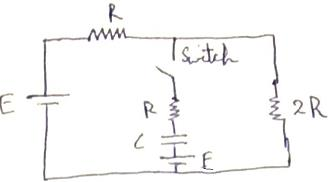Solution:
QUESTION: 12

A uniform rod of length L is charged uniformly with charge Q and is rotating about an axis passing through it's center and perpendicular to the rod with angular velcocity ω. Magnetic moment of the rod is,

Solution:
QUESTION: 13

Two SHM waves are represented by the equations, y1 = 6 cos(6πt + π/6) and y2 = 3(√3 sin 3πt + cos 3πt). Which of the following statements is correct,

Solution:
QUESTION: 14

The direction of field B at a point P* symmetric to point P with respect to the vertex, i.e, along the axis and the same distance d but inside the V region is along,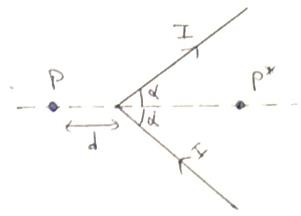Solution:
QUESTION: 15

A parallel plate capacitor without any dielectric has capacitance Co. A dielectric slab is made up of two dielectric slabs of dielectric constants K and 2K and is of same dimensions as that of capacitor plates and both parts are of equal dimensions areeanged in series. If this dielectric slab is introduced (with dielectric K enetering first) in between the plates at a constant speed, then the variation of capacitance with time is best represented by,

Solution:
QUESTION: 16

Two identical spheres of same mass and specific gravity (which is the ratio of density of a substance and density of water) 2.4 have different charges Q and -3Q. They are suspended from two strings of same length L fixed to points at the same horizontal level, but distance L from each other. When the entire set up is transferred inside a liquid of specific gravity 0.8, it is observed that the inclination of each string in equilibrium remains unchanged. Then the dielectric constant of the liquid is,

Solution:
QUESTION: 17

There are three concentric thin spheres of radius a,b,c (in decreasing order). The total surface charge densities on their surfaces are σ, -σ, σ respectively. The magnitude of electric field at a distance r from the center such that a > r > b is,

Solution:

Electric field at a distance r (a > r > b) will be due to charges enclosed in r only, & Since, a sphere acts as a point charge for points outside its surface,

∴ E = kQc/r2 + kQb/r2 = kr2

(σ×4πc+ (−σ)4πb2)

= σε0r2(c2− b2)

QUESTION: 18

A fixed container is fitted with a piston which is attached to a spring constant k. The other end of the spring is attached to a rigid wall. Initially the spring is in it's natural length and the length of the container is L. Now an ideal diatomic gas is slowly filled in the container so that the spring now is compressed by x. The total rotational kinetic energy of the gas molecules in terms of the displacement x of the piston is (there is vaccum outside the container),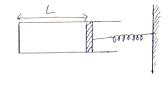Solution:
QUESTION: 19

A object is moving with velocity v (with respect ground) parallel to plane mirror M1. Another plane mirror M2 makes an angle β with the vertical as shown. Then the velocity of image in mirror M2 with respect to the image in M1 is,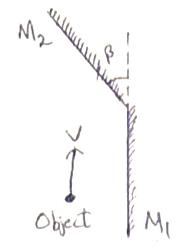Solution:

we have to find magnitude of relative velocity between v1 and v2.The angle between these two vectors is

Hence vr=v1−v2

|vr|=|v1−v2|
This is nothing but magnitude of subtration of two velocity vectors of equal magnitudes v each and angle between them equal to 2β
Hence

∣vr∣ = √v+ v+ 2(v)(v)cos2θ

Solving these two equations ,we get

∣v2∣ = 2v sinβ

QUESTION: 20

M1 and M2 are plane mirrors and kept parallel to each other. At point O there will be a maxima for wavelength. Light from monochromatic source S of wavelength λ is not reaching directly on the screen. Then λ is, (D >> d, d >> λ)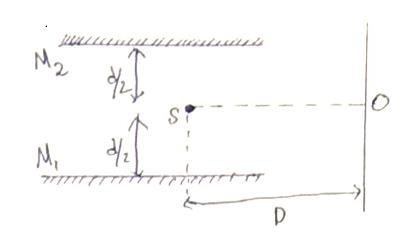Solution: